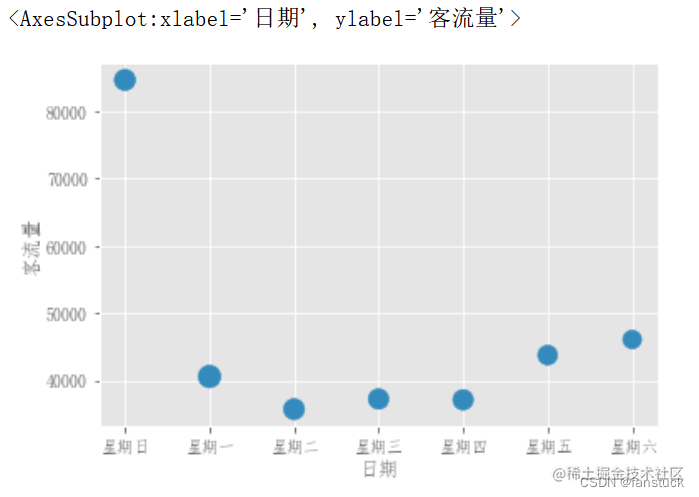# 前言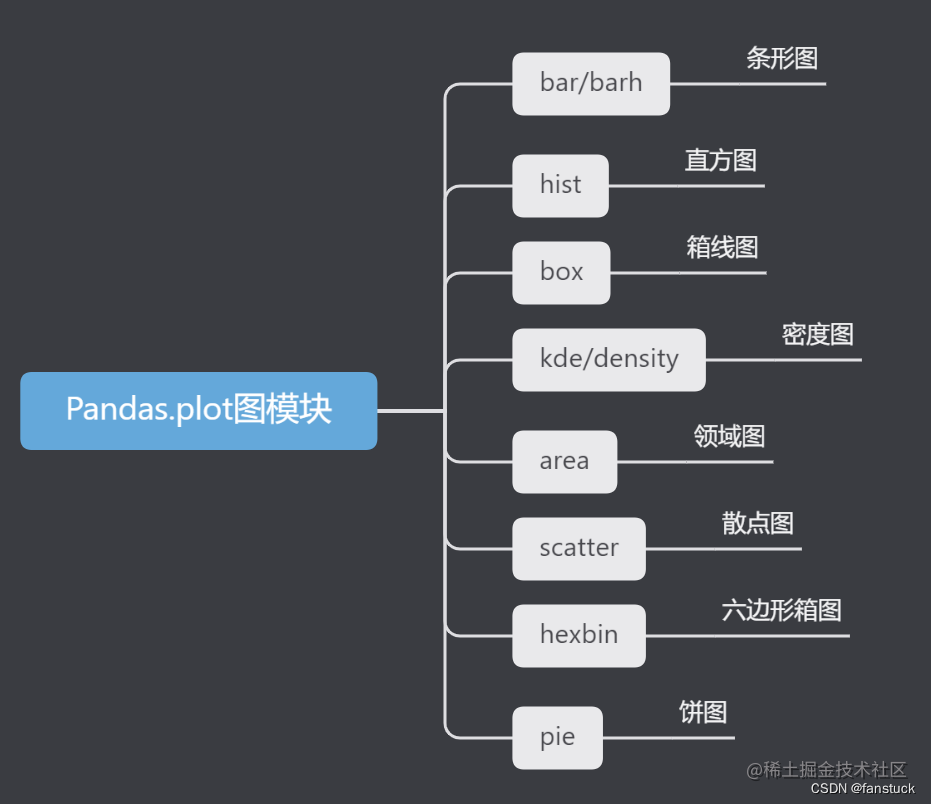Pandas数据分析系列专栏已经更新了很久了，基本覆盖到使用pandas处理日常业务以及常规的数据分析方方面面的问题。从基础的数据结构逐步入门到处理各类数据以及专业的pandas常用函数讲解都花费了大量时间和心思创作，如果大家有需要从事数据分析或者大数据开发的朋友推荐订阅专栏，将在第一时间学习到Pandas数据分析最实用常用的知识。此篇博客篇幅较长，涉及到数据可视化等各类操作，值得细读实践一番，我会将Pandas的精华部分挑出细讲实践。博主会长期维护博文，有错误或者疑惑可以在评论区指出，感谢大家的支持。

# 一、箱线图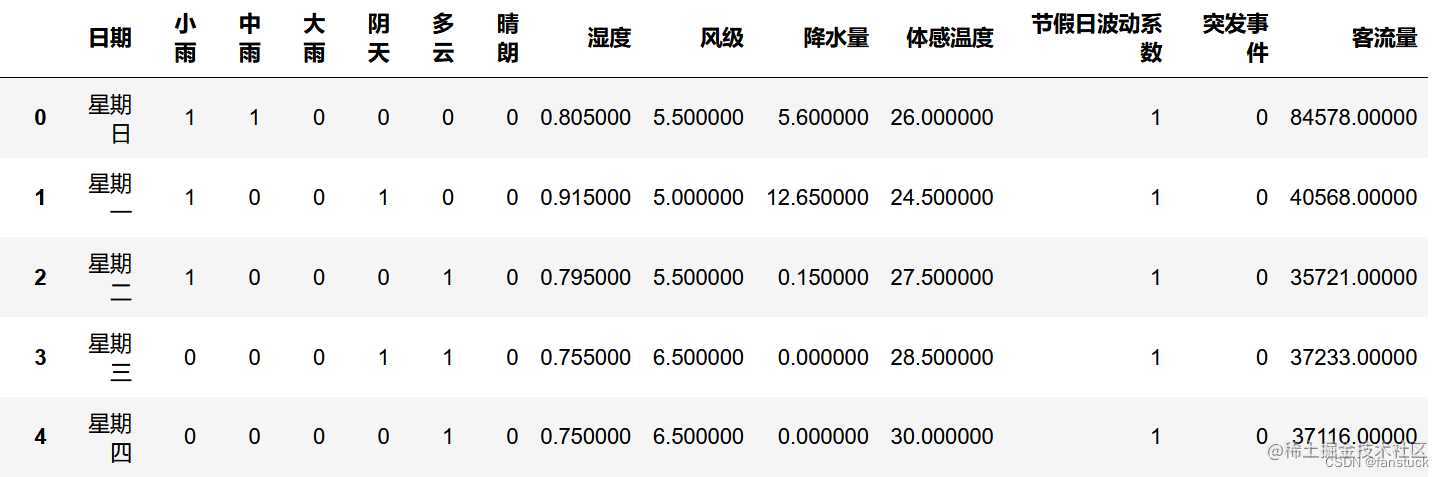• Series.plot.box()
• DataFrame.plot.box()
• DataFrame.boxplot()

`df_flow_mark[['风级','降水量']].plot.box()`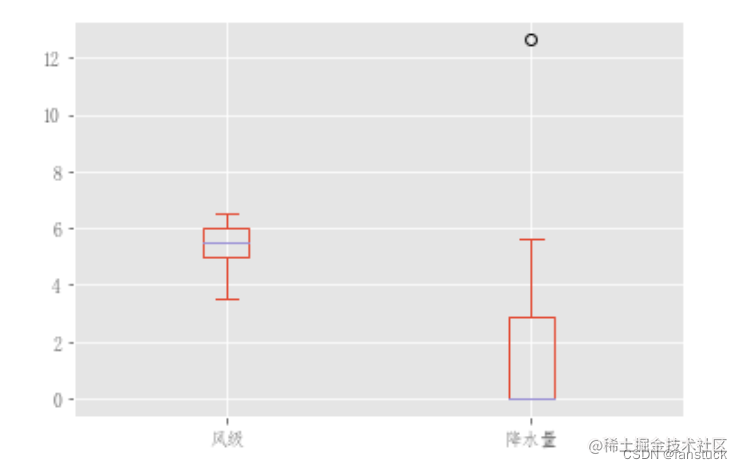可以通过传递color关键字对Boxplot进行着色。你可以传递一个字典dict，key关键字为boxes、whiskers,medians,caps。如果dict中缺少一些键，则会为相应的使用默认颜色。此外，箱线图还有sym关键字来指定传单样式。

```color = { "boxes": "DarkGreen", "whiskers": "DarkOrange", "medians": "DarkBlue", "caps": "Gray", } df_flow_mark[['风级','降水量']].plot.box(color=color, sym="r+")```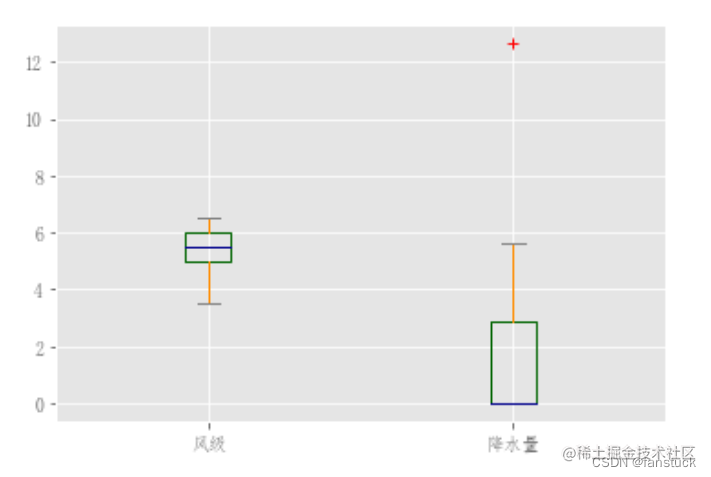创建一个数据集展示更加明显：

```df = pd.DataFrame(np.random.rand(10, 5), columns=["A", "B", "C", "D", "E"]) color = { "boxes": "DarkGreen", "whiskers": "DarkOrange", "medians": "DarkBlue", "caps": "Gray", } df.plot.box(color=color, sym="r+")```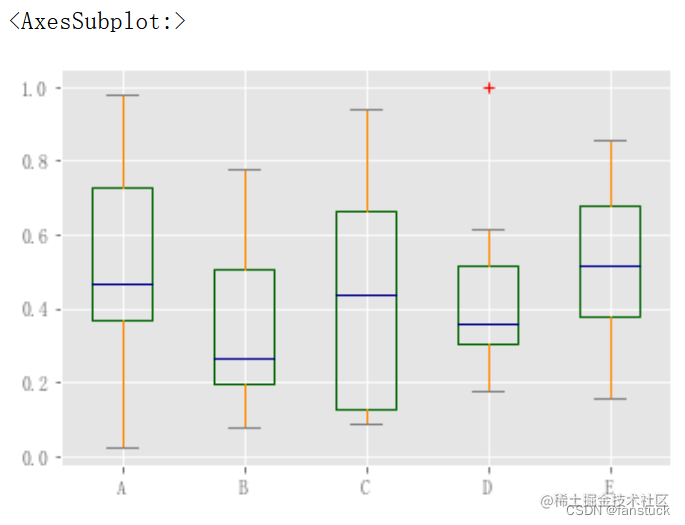此外，还可以传递matplotlib箱线图支持的其他关键字。例如，可以通过vert=False和positions关键字绘制水平和自定义定位箱线图。

`df.plot.box(vert=False, positions=[1, 4, 5, 6, 8])`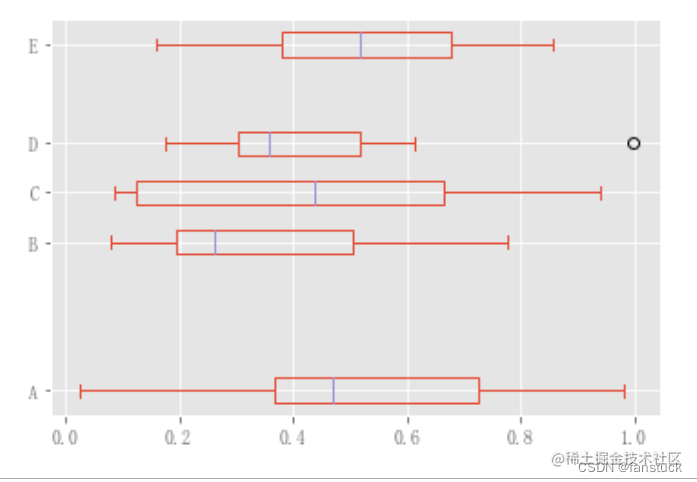现有接口仍然可以使用DataFrame.boxplot：

`df.boxplot()`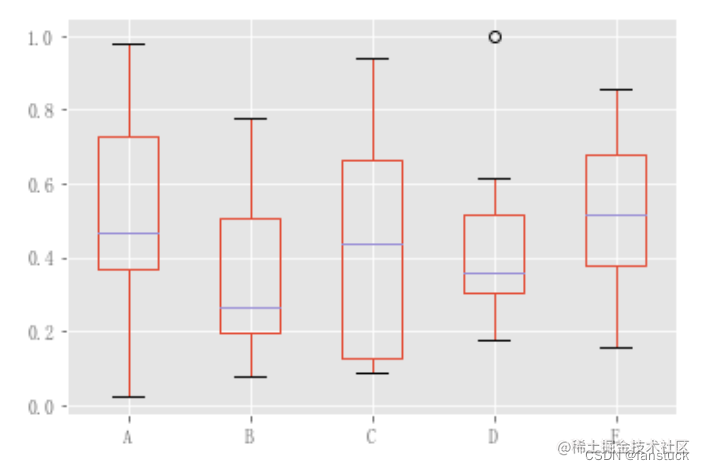可以使用by关键字参数创建分层箱线图来创建分组。例如，

```df = pd.DataFrame(np.random.rand(10, 2), columns=["Col1", "Col2"]) df["X"] = pd.Series(["A", "A", "A", "A", "A", "B", "B", "B", "B", "B"]) plt.figure(); bp = df.boxplot(by="X")```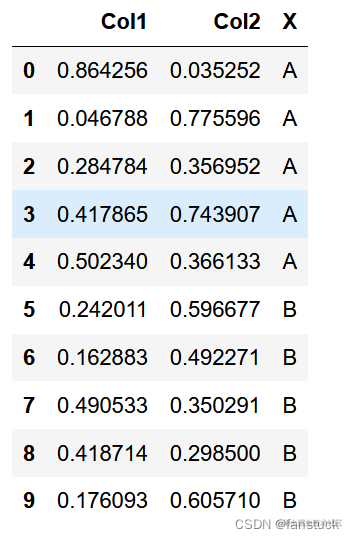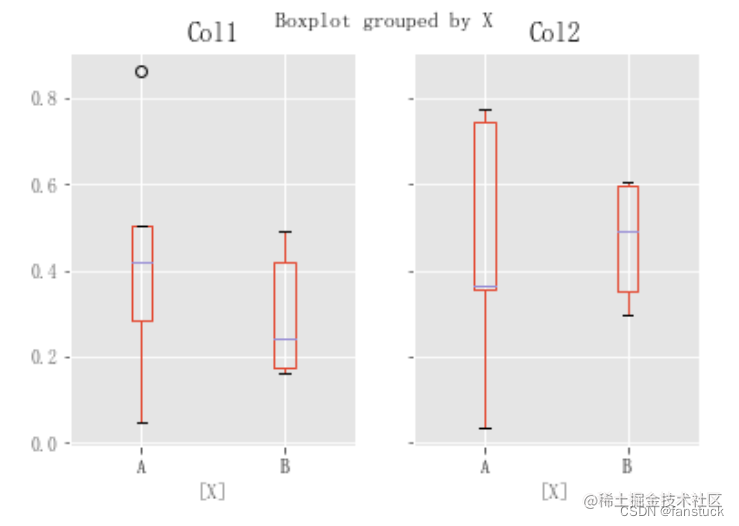还可以传递要打印的列子集，以及按多个列分组：

```df = pd.DataFrame(np.random.rand(10, 3), columns=["Col1", "Col2", "Col3"]) df["X"] = pd.Series(["A", "A", "A", "A", "A", "B", "B", "B", "B", "B"]) df["Y"] = pd.Series(["A", "B", "A", "B", "A", "B", "A", "B", "A", "B"]) plt.figure(); bp = df.boxplot(column=["Col1", "Col2"], by=["X", "Y"])```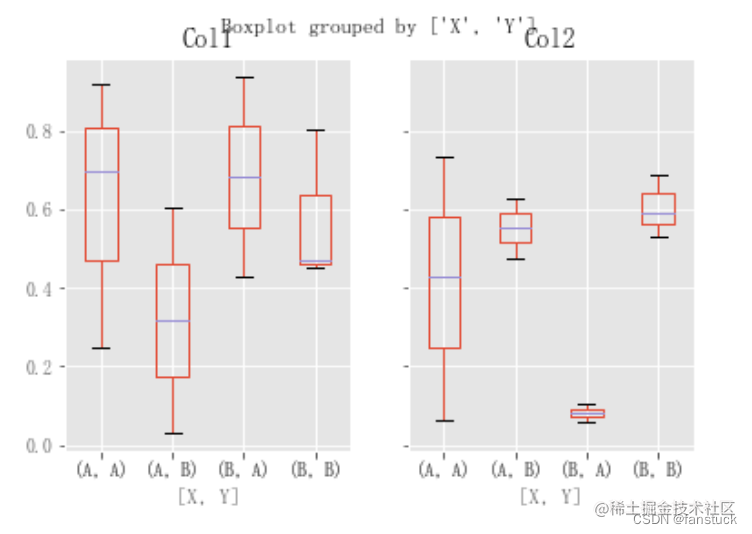用DataFrame.plot.box()也是一样的：

```df = pd.DataFrame(np.random.rand(10, 3), columns=["Col1", "Col2", "Col3"]) df["X"] = pd.Series(["A", "A", "A", "A", "A", "B", "B", "B", "B", "B"]) plt.figure() bp = df.plot.box(columns=["Col1", "Col2"], by="X")``` 在箱线图中，返回类型可以由return_type，关键字控制。有效选项是{“axes”、“dict”、“both”、“None}。镶嵌面，由DataFrame.boxplot创建by关键字的箱线图也会影响输出类型：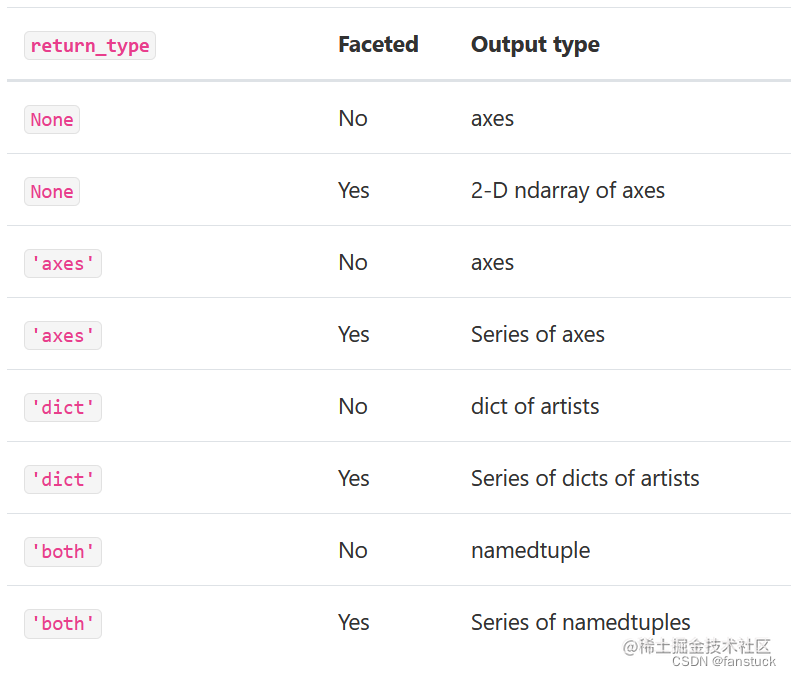```np.random.seed(1234) df_box = pd.DataFrame(np.random.randn(50, 2)) df_box["g"] = np.random.choice(["A", "B"], size=50) df_box.loc[df_box["g"] == "B", 1] += 3 bp = df_box.boxplot(by="g")```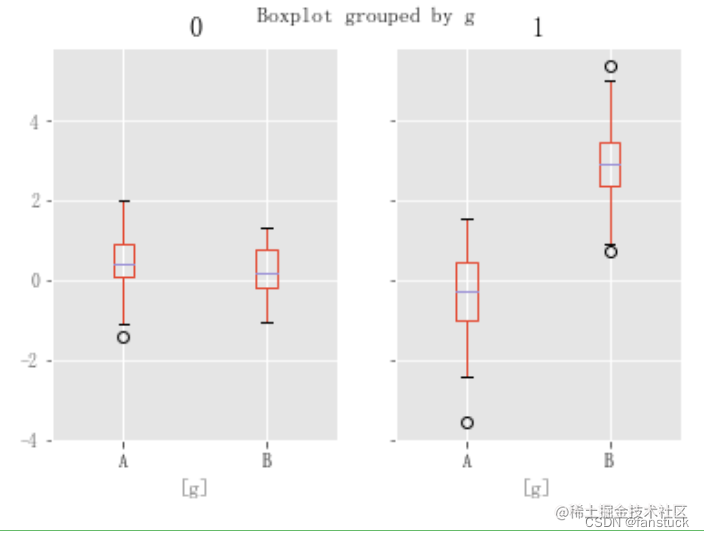上面的子地块首先由数字列分割，然后由g列的值分割。下面的子地块首先由g值分割，然后由数字列分割。

`bp = df_box.groupby("g").boxplot()`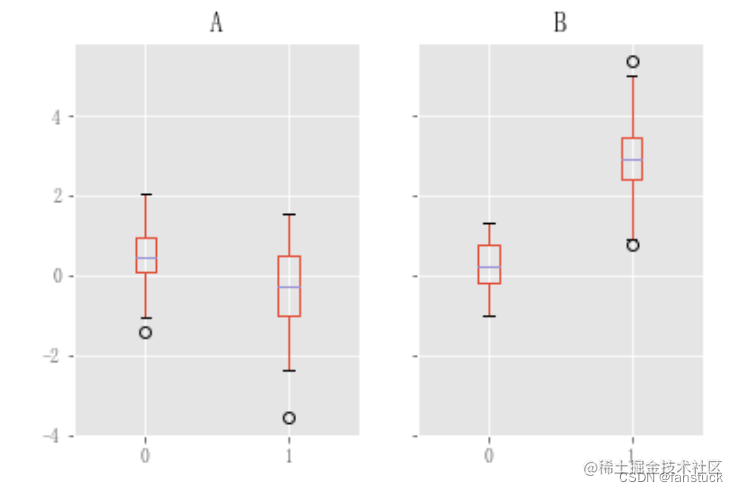# 二、面积填充图

```df = pd.DataFrame(np.random.rand(10, 4), columns=["a", "b", "c", "d"]) df.plot.area();```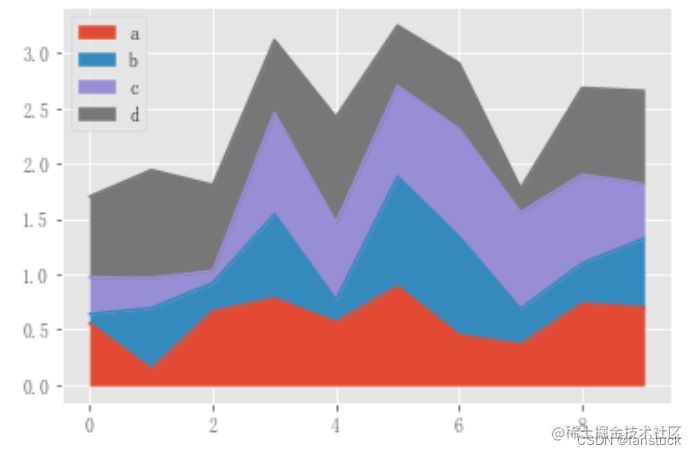要生成未堆叠的绘图，请传递stacked=False。Alpha值设置为0.5。

`df.plot.area(stacked=False);`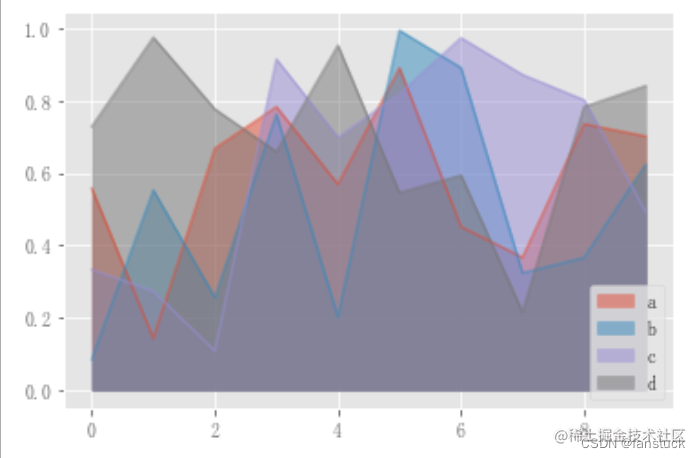# 三、散点图

`df_flow_mark.plot.scatter(x='日期',y='客流量')`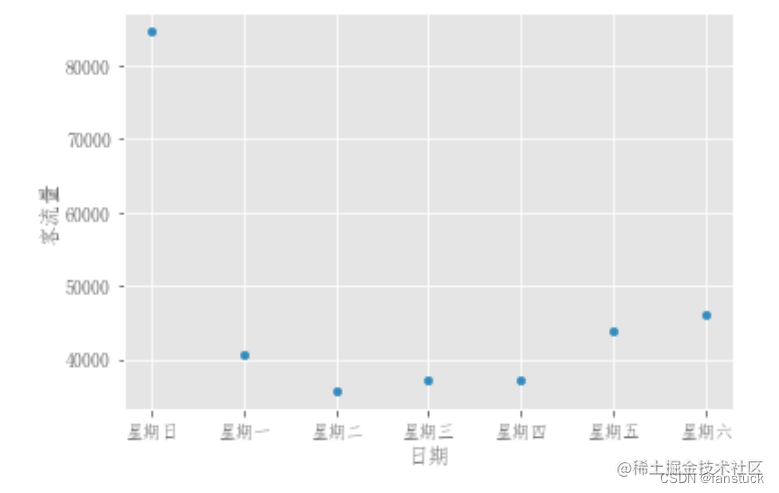要在单个轴上绘制多个列组，可以重复指定目标轴的打印方法。建议指定颜色(color)和标签(label)关键字以区分每个组。

```df = pd.DataFrame(np.random.rand(50, 4), columns=["a", "b", "c", "d"]) df["species"] = pd.Categorical( ["setosa"] * 20 + ["versicolor"] * 20 + ["virginica"] * 10 ) ax = df.plot.scatter(x="a", y="b", color="DarkBlue", label="Group 1") df.plot.scatter(x="c", y="d", color="DarkGreen", label="Group 2", ax=ax);```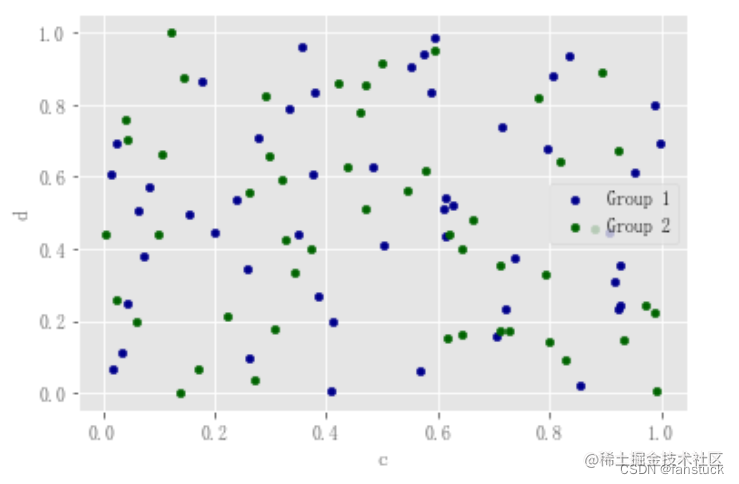关键字c可以作为列的名称，为每个点提供颜色：

```

df.plot.scatter(x="a", y="b", c="c", s=50); ```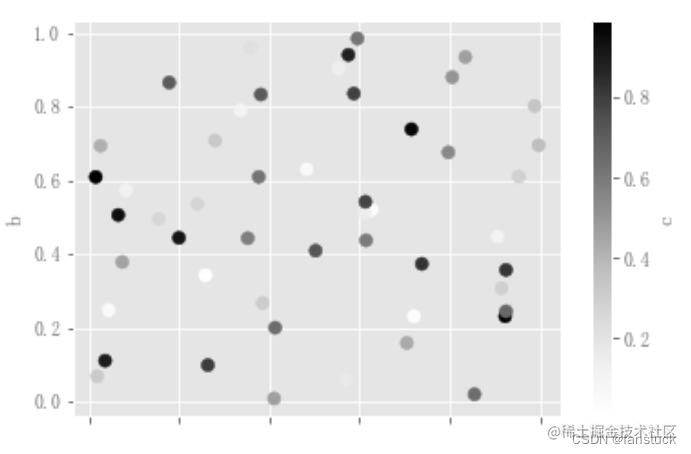如果将分类列传递给c，则将生成一个离散的颜色条：

`df.plot.scatter(x="a", y="b", c="species", cmap="viridis", s=50);`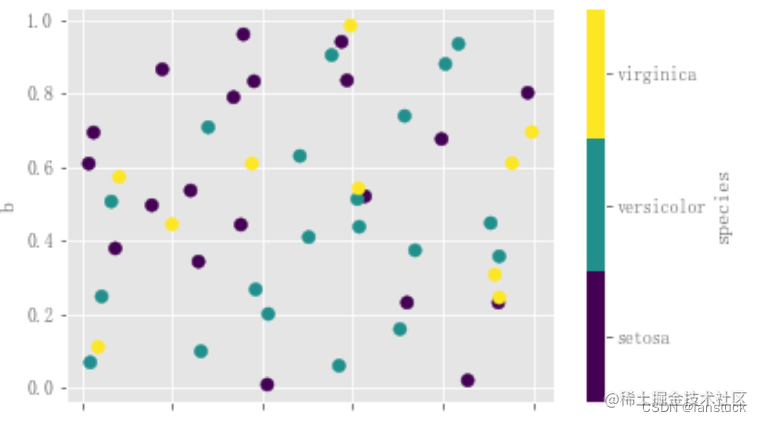可以传递matplotlib.scatter支持的其他关键字。下面的示例显示了一个气泡图，它使用DataFrame的一列作为气泡大小。

`df_flow_mark.plot.scatter(x='日期',y='客流量',s=df_flow_mark['湿度']*200)`